# High School Math : Circles

## Example Questions

← Previous 1 3 4 5 6 7 8 9 12 13

### Example Question #1 : How To Find The Angle For A Percentage Of A Circle

A sector comprises 20% of a circle. What is the central angle of the sector?Explanation:

Proporations can be used to solve for the central angle. Letequal the angle of the sector.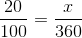Cross mulitply: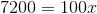Solve for: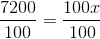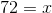### Example Question #2 : How To Find The Angle For A Percentage Of A Circle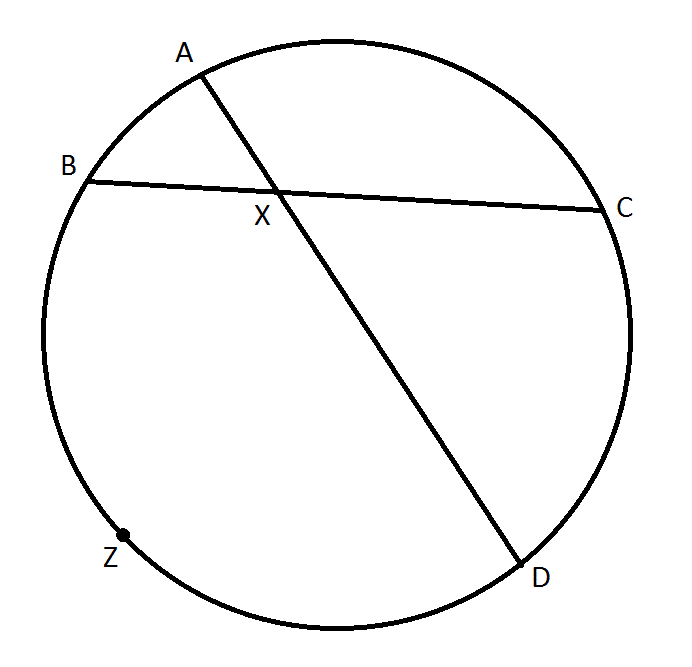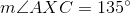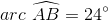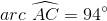Find the degree measure of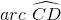.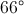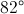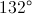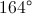Not enough information is given to answer this question.Explanation:

When two chords of a circle intersect, the measure of the angle they form is half the sum of the measures of the arcs they intercept. Therefore,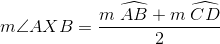Since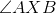and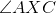form a linear pair,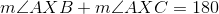, and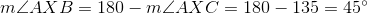.

Substitute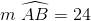and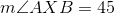into the first equation: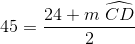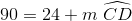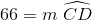### Example Question #1 : Sectors

Two pizzas are made to the same dimensions. The only difference is that Pizza 1 is cut into pieces at 30° angles and Pizza 2 is cut at 45° angles. They are sold by the piece, the first for $1.95 per slice and the second for$2.25 per slice. What is the difference in total revenue between Pizza 2 and Pizza 1?

$2.70$5.40

–$2.70$0

–$5.40 Correct answer: –$5.40

Explanation:

First, let's calculate how many slices there are per pizza. This is done by dividing 360° by the respective slice degrees:

Pizza 1: 360/30 = 12 slices

Pizza 2: 360/45 = 8 slices

Now, the total amount made per pizza is calculated by multiplying the number of slices by the respective cost per slice:

Pizza 1: 12 * 1.95 = $23.40 Pizza 2: 8 * 2.25 =$18.00

The difference between Pizza 2 and Pizza 1 is thus represented by: 18 – 23.40 = –\$5.40

### Example Question #1 : Circles

What percentage of a full circle is the following sector? (Round to the nearest tenth of a percent.)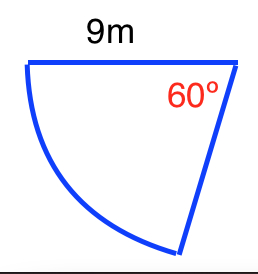Note: The figure is not drawn to scale.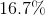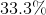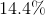Explanation:

In order to find the percentage of a sector from an angle, you need to know that a full circle is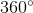.

Therefore, we can find the percentage by dividing the angle of the sector byand then multiplying by 100: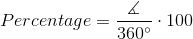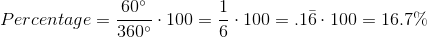### Example Question #2 : Circles

A sector of a circle contains a center angle that is 36 degrees. What percentage of the circle is the sector?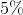Explanation:

Proportions can be used to determine the percentage. Letequal the percentage comprised of the sector.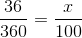Cross multiply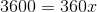Solve for x.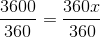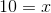### Example Question #1 : How To Find The Area Of A Sector

A circular, 8-slice pizza is placed in a square box that has dimensions four inches larger than the diameter of the pizza. If the box covers a surface area of 256 in2, what is the surface area of one piece of pizza?

4.5π in2

36π in2

144π in2

9π in2

18π in2

4.5π in2

Explanation:

The first thing to do is calculate the dimensions of the pizza box. Based on our data, we know 256 = s2. Solving for s (by taking the square root of both sides), we get 16 = s (or s = 16).

Now, we know that the diameter of the pizza is four inches less than 16 inches. That is, it is 12 inches. Be careful! The area of the circle is given in terms of radius, which is half the diameter, or 6 inches. Therefore, the area of the pizza is π * 62 = 36π in2. If the pizza is 8-slices, one slice is equal to 1/8 of the total pizza or (36π)/8 = 4.5π in2.

### Example Question #1 : How To Find The Area Of A Sector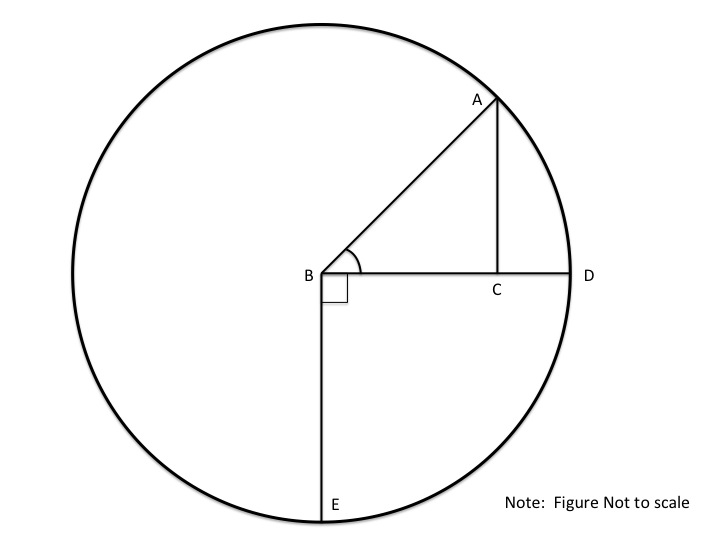If B is a circle with line AC = 12 and line BC = 16, then what is the area formed by DBE?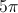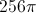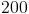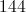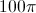Explanation:

Line AB is a radius of Circle B, which can be found using the Pythagorean Theorem: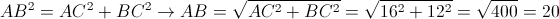Since AB is a radius of B, we can find the area of circle B via: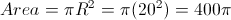Angle DBE is a right angle, and therefore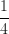of the circle so it follows: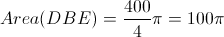### Example Question #1 : Circles

To the nearest tenth, give the area of asector of a circle with diameter 18 centimeters.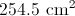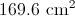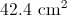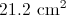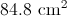Explanation:

The radius of a circle with diameter 18 centimeters is half that, or 9 centimeters. The area of asector of the circle is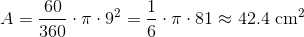### Example Question #162 : High School Math

Find the area of a sector that has an angle of 120 degrees and radius of 3.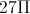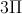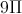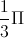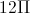Explanation:

The equation to find the area of a sector is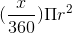.

Substitute the given radius in forand the given angle in forto get: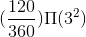Simplify the equation to get the area: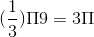### Example Question #163 : High School Math

What is the area of the following sector of a full circle?Note: Figure is not drawn to scale.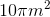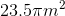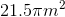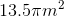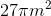Explanation:

In order to find the fraction of a sector from an angle, you need to know that a full circle is.

Therefore, we can find the fraction by dividing the angle of the sector by: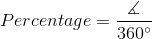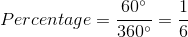The formula to find the area of a sector is: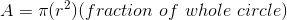whereis the radius of the circle.

Plugging in our values, we get: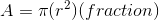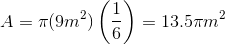← Previous 1 3 4 5 6 7 8 9 12 13